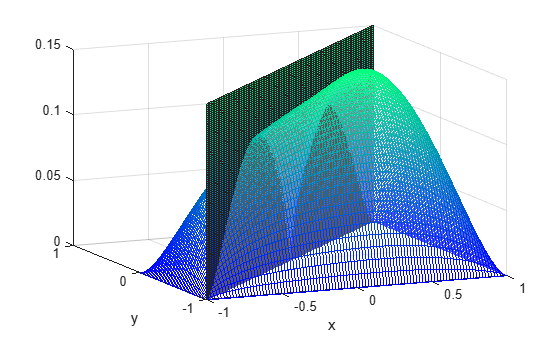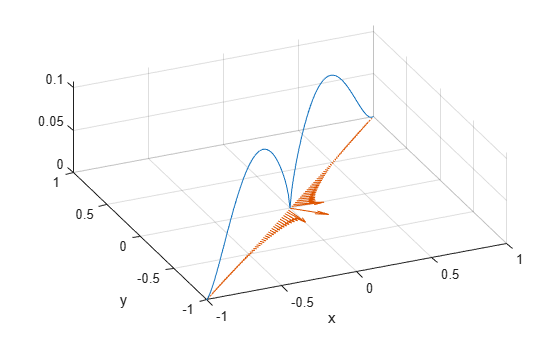Main Content

# 2-D Solution and Gradient Plots with MATLAB® Functions

You can interpolate the solution and, if needed, its gradient in separate steps, and then plot the results by using MATLAB® functions, such as `surf`, `mesh`, `quiver`, and so on. For example, solve the same scalar elliptic problem $-\Delta u=1$ on the L-shaped membrane with zero Dirichlet boundary conditions. Interpolate the solution and its gradient, and then plot the results.

Create the PDE model, 2-D geometry, and mesh. Specify boundary conditions and coefficients. Solve the PDE problem.

```model = createpde; geometryFromEdges(model,@lshapeg); applyBoundaryCondition(model,'dirichlet','edge',1:model.Geometry.NumEdges,'u',0); c = 1; a = 0; f = 1; specifyCoefficients(model,'m',0,'d',0,'c',c,'a',a,'f',f); generateMesh(model,'Hmax',0.05); results = solvepde(model);```

Interpolate the solution and its gradients to a dense grid from -1 to 1 in each direction.

```v = linspace(-1,1,101); [X,Y] = meshgrid(v); querypoints = [X(:),Y(:)]'; uintrp = interpolateSolution(results,querypoints);```

Plot the resulting solution on a mesh.

```uintrp = reshape(uintrp,size(X)); mesh(X,Y,uintrp) xlabel('x') ylabel('y')```Interpolate gradients of the solution to the grid from -1 to 1 in each direction. Plot the result using `quiver`.

```[gradx,grady] = evaluateGradient(results,querypoints); figure quiver(X(:),Y(:),gradx,grady) xlabel('x') ylabel('y')```

Zoom in to see more details. For example, restrict the range to `[-0.2,0.2]` in each direction.

`axis([-0.2 0.2 -0.2 0.2])`Plot the solution and the gradients on the same range.

```figure h1 = meshc(X,Y,uintrp); set(h1,'FaceColor','g','EdgeColor','b') xlabel('x') ylabel('y') alpha(0.5) hold on Z = -0.05*ones(size(X)); gradz = zeros(size(gradx)); h2 = quiver3(X(:),Y(:),Z(:),gradx,grady,gradz); set(h2,'Color','r') axis([-0.2,0.2,-0.2,0.2])```Slice of the solution plot along the line `x = y`.

```figure mesh(X,Y,uintrp) xlabel('x') ylabel('y') alpha(0.25) hold on z = linspace(0,0.15,101); Z = meshgrid(z); surf(X,X,Z') view([-20 -45 15]) colormap winter```Plot the interpolated solution along the line.

```figure xq = v; yq = v; uintrp = interpolateSolution(results,xq,yq); plot3(xq,yq,uintrp) grid on xlabel('x') ylabel('y')```

Interpolate gradients of the solution along the same line and add them to the solution plot.

```[gradx,grady] = evaluateGradient(results,xq,yq); gradx = reshape(gradx,size(xq)); grady = reshape(grady,size(yq)); hold on quiver(xq,yq,gradx,grady) view([-20 -45 75])```## SupportGet trial now